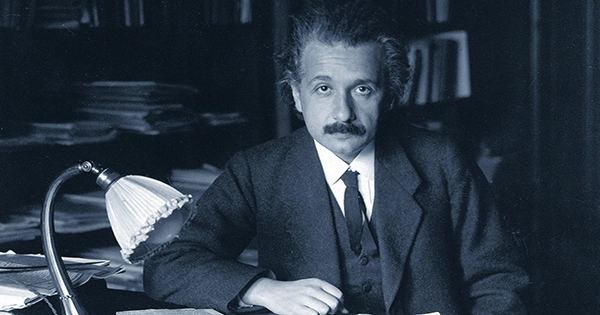Physics

# Albert Einstein Describes His Most Famous Equation in a VideoOn Twitter, a video of Albert Einstein discussing his most well-known physics equation—possibly the most well-known equation—has received a lot of attention.

In the video, Einstein explains the equation E=MC2, which expresses the equivalence of mass and energy.

According to the special theory of relativity, “mass and energy are both just different expressions of the same thing,” as Einstein explains in the video, “a somewhat foreign thought for the normal mind.

“Furthermore, it was demonstrated that extremely small amounts of mass may be transformed into very enormous amounts of energy and vice versa using the equation E is equal to m c-squared, in which energy is set equal to mass, multiplied by the square of the velocity of light. The aforementioned equation showed that the mass and energy were indeed equal. In 1932, Cockcroft and Walton carried out an experimental demonstration of this.”

He was referring to an experiment in which John Cockcroft and Ernest Walton used a proton beam to irradiate lithium and beryllium targets. The team’s goal was to break up atom nuclei, which they successfully accomplished by splitting lithium into two helium nuclei. As an added bonus, they discovered that the total kinetic energy of the newly formed helium nuclei was higher than that of the original hydrogen and lithium nuclei and that there had been a commensurate loss of mass, so verifying the equation E=MC2.

They received a Nobel Prize in Physics in 1951 after conducting their experiment repeatedly with many other atomic nuclei.